# 8.4 Polar coordinates: graphs  (Page 7/16)

 Page 7 / 16

Sketch the graph of $\text{\hspace{0.17em}}r=-\theta \text{\hspace{0.17em}}$ over the interval $\text{\hspace{0.17em}}\left[0,4\pi \right].$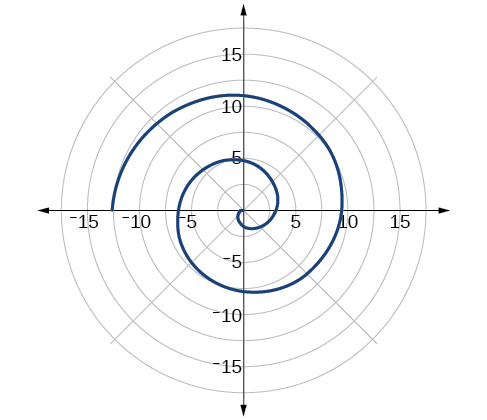## Summary of curves

We have explored a number of seemingly complex polar curves in this section. [link] and [link] summarize the graphs and equations for each of these curves.

Access these online resources for additional instruction and practice with graphs of polar coordinates.

## Key concepts

• It is easier to graph polar equations if we can test the equations for symmetry with respect to the line $\text{\hspace{0.17em}}\theta =\frac{\pi }{2},\text{\hspace{0.17em}}$ the polar axis, or the pole.
• There are three symmetry tests that indicate whether the graph of a polar equation will exhibit symmetry. If an equation fails a symmetry test, the graph may or may not exhibit symmetry. See [link] .
• Polar equations may be graphed by making a table of values for $\text{\hspace{0.17em}}\theta \text{\hspace{0.17em}}$ and $\text{\hspace{0.17em}}r.$
• The maximum value of a polar equation is found by substituting the value $\text{\hspace{0.17em}}\theta \text{\hspace{0.17em}}$ that leads to the maximum value of the trigonometric expression.
• The zeros of a polar equation are found by setting $\text{\hspace{0.17em}}r=0\text{\hspace{0.17em}}$ and solving for $\text{\hspace{0.17em}}\theta .\text{\hspace{0.17em}}$ See [link] .
• Some formulas that produce the graph of a circle in polar coordinates are given by $\text{\hspace{0.17em}}r=a\mathrm{cos}\text{\hspace{0.17em}}\theta \text{\hspace{0.17em}}$ and $\text{\hspace{0.17em}}r=a\mathrm{sin}\text{\hspace{0.17em}}\theta .\text{\hspace{0.17em}}$ See [link] .
• The formulas that produce the graphs of a cardioid are given by $\text{\hspace{0.17em}}r=a±b\mathrm{cos}\text{\hspace{0.17em}}\theta \text{\hspace{0.17em}}$ and $\text{\hspace{0.17em}}r=a±b\mathrm{sin}\text{\hspace{0.17em}}\theta ,\text{\hspace{0.17em}}$ for $\text{\hspace{0.17em}}a>0,\text{\hspace{0.17em}}\text{\hspace{0.17em}}b>0,\text{\hspace{0.17em}}$ and $\text{\hspace{0.17em}}\frac{a}{b}=1.\text{\hspace{0.17em}}$ See [link] .
• The formulas that produce the graphs of a one-loop limaçon are given by $\text{\hspace{0.17em}}r=a±b\mathrm{cos}\text{\hspace{0.17em}}\theta \text{\hspace{0.17em}}$ and $\text{\hspace{0.17em}}r=a±b\mathrm{sin}\text{\hspace{0.17em}}\theta \text{\hspace{0.17em}}$ for $\text{\hspace{0.17em}}1<\frac{a}{b}<2.\text{\hspace{0.17em}}$ See [link] .
• The formulas that produce the graphs of an inner-loop limaçon are given by $\text{\hspace{0.17em}}r=a±b\mathrm{cos}\text{\hspace{0.17em}}\theta \text{\hspace{0.17em}}$ and $\text{\hspace{0.17em}}r=a±b\mathrm{sin}\text{\hspace{0.17em}}\theta \text{\hspace{0.17em}}$ for $\text{\hspace{0.17em}}a>0,\text{\hspace{0.17em}}\text{\hspace{0.17em}}b>0,\text{\hspace{0.17em}}$ and $\text{\hspace{0.17em}}a See [link] .
• The formulas that produce the graphs of a lemniscates are given by $\text{\hspace{0.17em}}{r}^{2}={a}^{2}\mathrm{cos}\text{\hspace{0.17em}}2\theta \text{\hspace{0.17em}}$ and $\text{\hspace{0.17em}}{r}^{2}={a}^{2}\mathrm{sin}\text{\hspace{0.17em}}2\theta ,\text{\hspace{0.17em}}$ where $\text{\hspace{0.17em}}a\ne 0.$ See [link] .
• The formulas that produce the graphs of rose curves are given by $\text{\hspace{0.17em}}r=a\mathrm{cos}\text{\hspace{0.17em}}n\theta \text{\hspace{0.17em}}$ and $\text{\hspace{0.17em}}r=a\mathrm{sin}\text{\hspace{0.17em}}n\theta ,\text{\hspace{0.17em}}$ where $\text{\hspace{0.17em}}a\ne 0;\text{\hspace{0.17em}}$ if $\text{\hspace{0.17em}}n\text{\hspace{0.17em}}$ is even, there are $\text{\hspace{0.17em}}2n\text{\hspace{0.17em}}$ petals, and if $\text{\hspace{0.17em}}n\text{\hspace{0.17em}}$ is odd, there are $\text{\hspace{0.17em}}n\text{\hspace{0.17em}}$ petals. See [link] and [link] .
• The formula that produces the graph of an Archimedes’ spiral is given by $\text{\hspace{0.17em}}r=\theta ,\text{\hspace{0.17em}}\text{\hspace{0.17em}}\theta \ge 0.\text{\hspace{0.17em}}$ See [link] .

## Verbal

Describe the three types of symmetry in polar graphs, and compare them to the symmetry of the Cartesian plane.

Symmetry with respect to the polar axis is similar to symmetry about the $\text{\hspace{0.17em}}x$ -axis, symmetry with respect to the pole is similar to symmetry about the origin, and symmetric with respect to the line $\text{\hspace{0.17em}}\theta =\frac{\pi }{2}\text{\hspace{0.17em}}$ is similar to symmetry about the $\text{\hspace{0.17em}}y$ -axis.

Which of the three types of symmetries for polar graphs correspond to the symmetries with respect to the x -axis, y -axis, and origin?

What are the steps to follow when graphing polar equations?

Test for symmetry; find zeros, intercepts, and maxima; make a table of values. Decide the general type of graph, cardioid, limaçon, lemniscate, etc., then plot points at and sketch the graph.

Describe the shapes of the graphs of cardioids, limaçons, and lemniscates.

What part of the equation determines the shape of the graph of a polar equation?

The shape of the polar graph is determined by whether or not it includes a sine, a cosine, and constants in the equation.

## Graphical

For the following exercises, test the equation for symmetry.

$r=5\mathrm{cos}\text{\hspace{0.17em}}3\theta$

$r=3-3\mathrm{cos}\text{\hspace{0.17em}}\theta$

symmetric with respect to the polar axis

$r=3+2\mathrm{sin}\text{\hspace{0.17em}}\theta$

$r=3\mathrm{sin}\text{\hspace{0.17em}}2\theta$

symmetric with respect to the polar axis, symmetric with respect to the line $\theta =\frac{\pi }{2},$ symmetric with respect to the pole

$r=4$

$r=2\theta$

no symmetry

$r=4\mathrm{cos}\text{\hspace{0.17em}}\frac{\theta }{2}$

$r=\frac{2}{\theta }$

no symmetry

$r=3\sqrt{1-{\mathrm{cos}}^{2}\theta }$

$r=\sqrt{5\mathrm{sin}\text{\hspace{0.17em}}2\theta }$

symmetric with respect to the pole

For the following exercises, graph the polar equation. Identify the name of the shape.

$r=3\mathrm{cos}\text{\hspace{0.17em}}\theta$

$r=4\mathrm{sin}\text{\hspace{0.17em}}\theta$

circle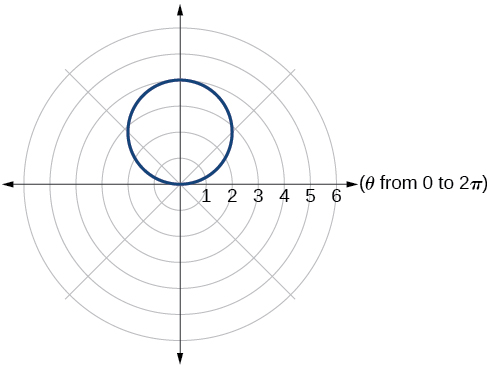$r=2+2\mathrm{cos}\text{\hspace{0.17em}}\theta$

$r=2-2\mathrm{cos}\text{\hspace{0.17em}}\theta$

cardioid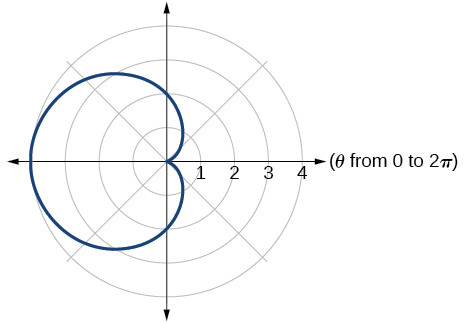$r=5-5\mathrm{sin}\text{\hspace{0.17em}}\theta$

$r=3+3\mathrm{sin}\text{\hspace{0.17em}}\theta$

cardioid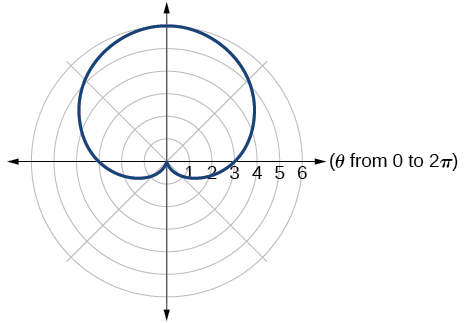$r=3+2\mathrm{sin}\text{\hspace{0.17em}}\theta$

$r=7+4\mathrm{sin}\text{\hspace{0.17em}}\theta$

one-loop/dimpled limaçon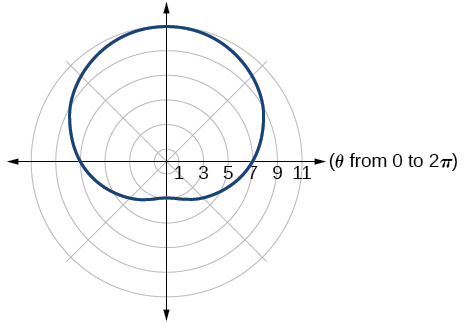$r=4+3\mathrm{cos}\text{\hspace{0.17em}}\theta$

$r=5+4\mathrm{cos}\text{\hspace{0.17em}}\theta$

one-loop/dimpled limaçon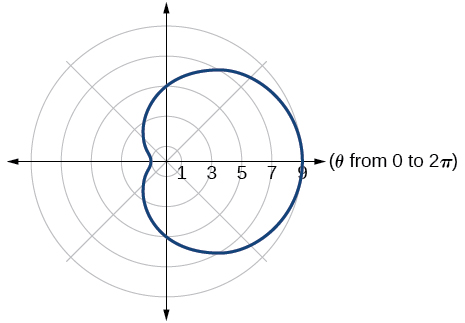$r=10+9\mathrm{cos}\text{\hspace{0.17em}}\theta$

$r=1+3\mathrm{sin}\text{\hspace{0.17em}}\theta$

inner loop/two-loop limaçon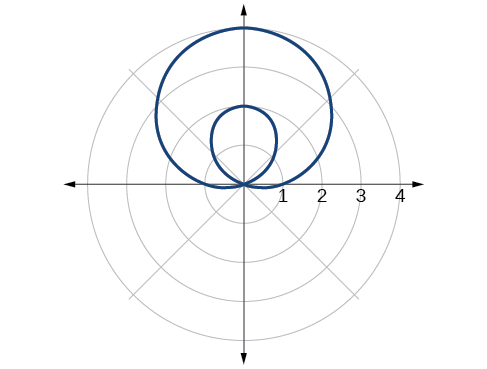$r=2+5\mathrm{sin}\text{\hspace{0.17em}}\theta$

$r=5+7\mathrm{sin}\text{\hspace{0.17em}}\theta$

inner loop/two-loop limaçon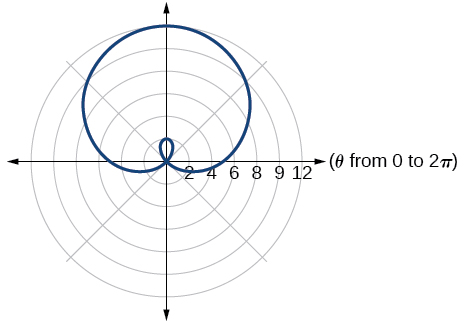$r=2+4\mathrm{cos}\text{\hspace{0.17em}}\theta$

$r=5+6\mathrm{cos}\text{\hspace{0.17em}}\theta$

inner loop/two-loop limaçon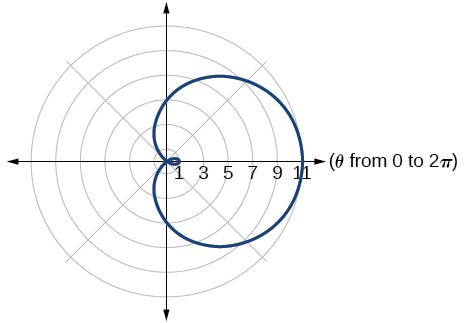${r}^{2}=36\mathrm{cos}\left(2\theta \right)$

${r}^{2}=10\mathrm{cos}\left(2\theta \right)$

lemniscate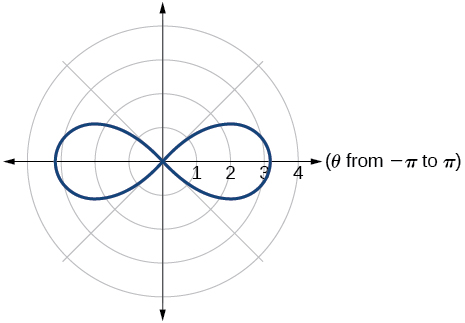${r}^{2}=4\mathrm{sin}\left(2\theta \right)$

${r}^{2}=10\mathrm{sin}\left(2\theta \right)$

lemniscate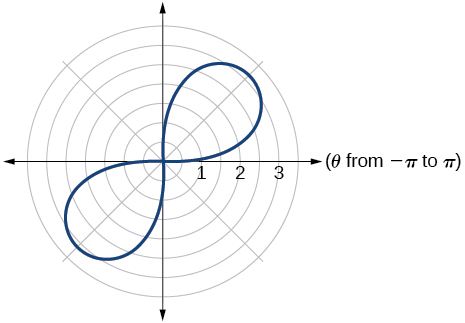$r=3\text{sin}\left(2\theta \right)$

$r=3\text{cos}\left(2\theta \right)$

rose curve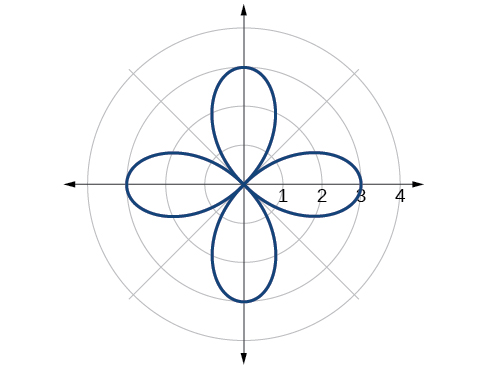$r=5\text{sin}\left(3\theta \right)$

$r=4\text{sin}\left(4\theta \right)$

rose curve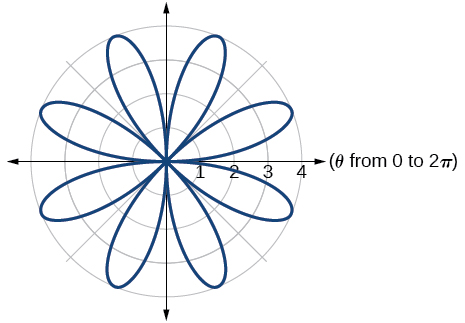$r=4\text{sin}\left(5\theta \right)$

$r=-\theta$

Archimedes’ spiral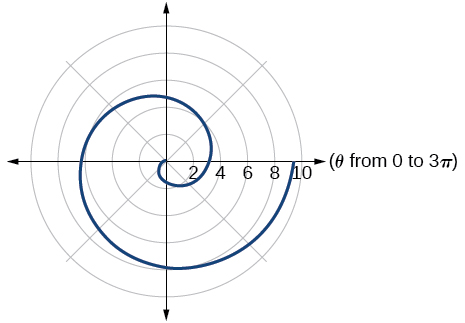$r=2\theta$

$r=-3\theta$

Archimedes’ spiral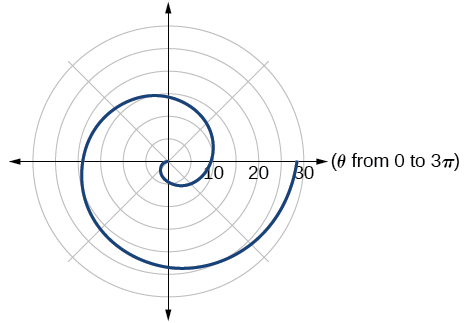## Technology

For the following exercises, use a graphing calculator to sketch the graph of the polar equation.

$r=\frac{1}{\theta }$

$r=\frac{1}{\sqrt{\theta }}$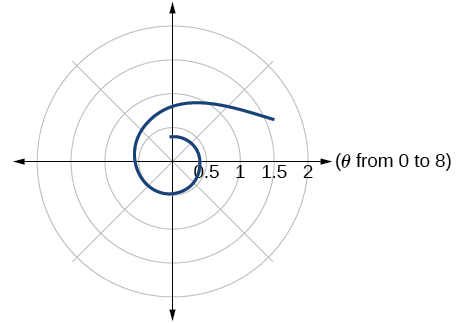$r=2\mathrm{sin}\text{\hspace{0.17em}}\theta \mathrm{tan}\text{\hspace{0.17em}}\theta ,$ a cissoid

$r=2\sqrt{1-{\mathrm{sin}}^{2}\theta }$ , a hippopede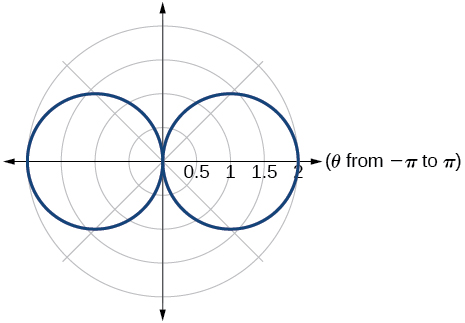$r=5+\mathrm{cos}\left(4\theta \right)$

$r=2-\mathrm{sin}\left(2\theta \right)$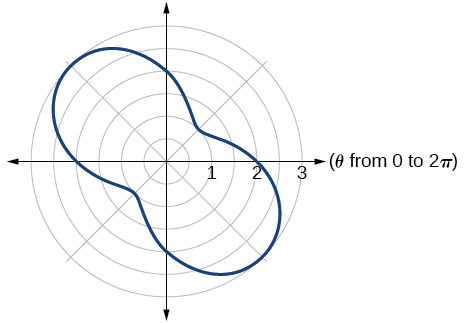$r={\theta }^{2}$

$r=\theta +1$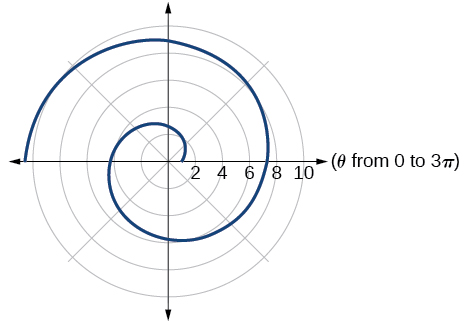$r=\theta \mathrm{sin}\text{\hspace{0.17em}}\theta$

$r=\theta \mathrm{cos}\text{\hspace{0.17em}}\theta$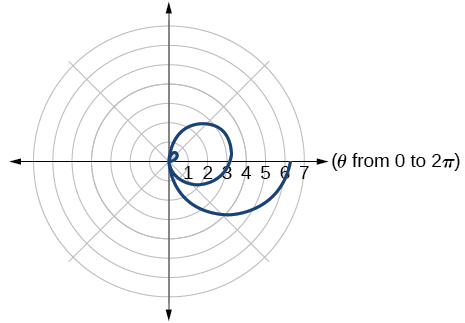For the following exercises, use a graphing utility to graph each pair of polar equations on a domain of $\text{\hspace{0.17em}}\left[0,4\pi \right]\text{\hspace{0.17em}}$ and then explain the differences shown in the graphs.

$r=\theta ,r=-\theta$

$r=\theta ,r=\theta +\mathrm{sin}\text{\hspace{0.17em}}\theta$

They are both spirals, but not quite the same.

$r=\mathrm{sin}\text{\hspace{0.17em}}\theta +\theta ,r=\mathrm{sin}\text{\hspace{0.17em}}\theta -\theta$

$r=2\mathrm{sin}\left(\frac{\theta }{2}\right),r=\theta \mathrm{sin}\left(\frac{\theta }{2}\right)$

Both graphs are curves with 2 loops. The equation with a coefficient of $\text{\hspace{0.17em}}\theta \text{\hspace{0.17em}}$ has two loops on the left, the equation with a coefficient of 2 has two loops side by side. Graph these from 0 to $\text{\hspace{0.17em}}4\pi \text{\hspace{0.17em}}$ to get a better picture.

$r=\mathrm{sin}\left(\mathrm{cos}\left(3\theta \right)\right)\text{\hspace{0.17em}}\text{\hspace{0.17em}}r=\mathrm{sin}\left(3\theta \right)$

On a graphing utility, graph $\text{\hspace{0.17em}}r=\mathrm{sin}\left(\frac{16}{5}\theta \right)\text{\hspace{0.17em}}$ on $\text{\hspace{0.17em}}\left[0,4\pi \right],\left[0,8\pi \right],\left[0,12\pi \right],\text{\hspace{0.17em}}$ and $\text{\hspace{0.17em}}\left[0,16\pi \right].\text{\hspace{0.17em}}$ Describe the effect of increasing the width of the domain.

When the width of the domain is increased, more petals of the flower are visible.

On a graphing utility, graph and sketch $\text{\hspace{0.17em}}r=\mathrm{sin}\text{\hspace{0.17em}}\theta +{\left(\mathrm{sin}\left(\frac{5}{2}\theta \right)\right)}^{3}\text{\hspace{0.17em}}$ on $\text{\hspace{0.17em}}\left[0,4\pi \right].$

On a graphing utility, graph each polar equation. Explain the similarities and differences you observe in the graphs.

$\begin{array}{l}\begin{array}{l}\\ {r}_{1}=3\mathrm{sin}\left(3\theta \right)\end{array}\hfill \\ {r}_{2}=2\mathrm{sin}\left(3\theta \right)\hfill \\ {r}_{3}=\mathrm{sin}\left(3\theta \right)\hfill \end{array}$

The graphs are three-petal, rose curves. The larger the coefficient, the greater the curve’s distance from the pole.

On a graphing utility, graph each polar equation. Explain the similarities and differences you observe in the graphs.

$\begin{array}{l}\begin{array}{l}\\ {r}_{1}=3+3\mathrm{cos}\text{\hspace{0.17em}}\theta \end{array}\hfill \\ {r}_{2}=2+2\mathrm{cos}\text{\hspace{0.17em}}\theta \hfill \\ {r}_{3}=1+\mathrm{cos}\text{\hspace{0.17em}}\theta \hfill \end{array}$

On a graphing utility, graph each polar equation. Explain the similarities and differences you observe in the graphs.

$\begin{array}{l}\begin{array}{l}\\ {r}_{1}=3\theta \end{array}\hfill \\ {r}_{2}=2\theta \hfill \\ {r}_{3}=\theta \hfill \end{array}$

The graphs are spirals. The smaller the coefficient, the tighter the spiral.

## Extensions

For the following exercises, draw each polar equation on the same set of polar axes, and find the points of intersection.

${r}_{1}=3+2\mathrm{sin}\text{\hspace{0.17em}}\theta ,\text{\hspace{0.17em}}{r}_{2}=2$

${r}_{1}=6-4\mathrm{cos}\text{\hspace{0.17em}}\theta ,\text{\hspace{0.17em}}{r}_{2}=4$

$\left(4,\frac{\pi }{3}\right),\left(4,\frac{5\pi }{3}\right)$

${r}_{1}=1+\mathrm{sin}\text{\hspace{0.17em}}\theta ,\text{\hspace{0.17em}}{r}_{2}=3\mathrm{sin}\text{\hspace{0.17em}}\theta$

${r}_{1}=1+\mathrm{cos}\text{\hspace{0.17em}}\theta ,\text{\hspace{0.17em}}{r}_{2}=3\mathrm{cos}\text{\hspace{0.17em}}\theta$

$\left(\frac{3}{2},\frac{\pi }{3}\right),\left(\frac{3}{2},\frac{5\pi }{3}\right)$

${r}_{1}=\mathrm{cos}\left(2\theta \right),\text{\hspace{0.17em}}{r}_{2}=\mathrm{sin}\left(2\theta \right)$

${r}_{1}={\mathrm{sin}}^{2}\left(2\theta \right),\text{\hspace{0.17em}}{r}_{2}=1-\mathrm{cos}\left(4\theta \right)$

$\left(0,\frac{\pi }{2}\right),\text{\hspace{0.17em}}\left(0,\pi \right),\text{\hspace{0.17em}}\left(0,\frac{3\pi }{2}\right),\text{\hspace{0.17em}}\left(0,2\pi \right)$

${r}_{1}=\sqrt{3},\text{\hspace{0.17em}}{r}_{2}=2\mathrm{sin}\left(\theta \right)$

${r}_{1}{}^{2}=\mathrm{sin}\text{\hspace{0.17em}}\theta ,{r}_{2}{}^{2}=\mathrm{cos}\text{\hspace{0.17em}}\theta$

$\left(\frac{\sqrt{8}}{2},\frac{\pi }{4}\right),\text{\hspace{0.17em}}\left(\frac{\sqrt{8}}{2},\frac{5\pi }{4}\right)\text{\hspace{0.17em}}$ and at $\text{\hspace{0.17em}}\theta =\frac{3\pi }{4},\text{\hspace{0.17em}}\text{\hspace{0.17em}}\frac{7\pi }{4}\text{\hspace{0.17em}}\text{\hspace{0.17em}}$ since $\text{\hspace{0.17em}}r\text{\hspace{0.17em}}$ is squared

${r}_{1}=1+\mathrm{cos}\text{\hspace{0.17em}}\theta ,\text{\hspace{0.17em}}{r}_{2}=1-\mathrm{sin}\text{\hspace{0.17em}}\theta$

how fast can i understand functions without much difficulty
what is set?
a colony of bacteria is growing exponentially doubling in size every 100 minutes. how much minutes will it take for the colony of bacteria to triple in size
I got 300 minutes. is it right?
Patience
no. should be about 150 minutes.
Jason
It should be 158.5 minutes.
Mr
ok, thanks
Patience
100•3=300 300=50•2^x 6=2^x x=log_2(6) =2.5849625 so, 300=50•2^2.5849625 and, so, the # of bacteria will double every (100•2.5849625) = 258.49625 minutes
Thomas
what is the importance knowing the graph of circular functions?
can get some help basic precalculus
What do you need help with?
Andrew
how to convert general to standard form with not perfect trinomial
can get some help inverse function
ismail
Rectangle coordinate
how to find for x
it depends on the equation
Robert
yeah, it does. why do we attempt to gain all of them one side or the other?
Melissa
whats a domain
The domain of a function is the set of all input on which the function is defined. For example all real numbers are the Domain of any Polynomial function.
Spiro
Spiro; thanks for putting it out there like that, 😁
Melissa
foci (–7,–17) and (–7,17), the absolute value of the differenceof the distances of any point from the foci is 24.
difference between calculus and pre calculus?
give me an example of a problem so that I can practice answering
x³+y³+z³=42
Robert
dont forget the cube in each variable ;)
Robert
of she solves that, well ... then she has a lot of computational force under her command ....
Walter
what is a function?
I want to learn about the law of exponent
explain this

#### Get Jobilize Job Search Mobile App in your pocket Now!ByBy Sheila LopezBy OpenStaxBy OpenStaxBy Jessica CollettBy Eddie UnverzagtBy OpenStaxBy OpenStaxBy JavaChamp TeamBy George TurnerBy OpenStax